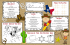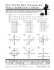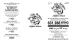# Why students of all ages are failing mathematics and

## Transcription

Why students of all ages are failing mathematics and
```Why students of all ages are failing mathematics and
what can be done to turn it around
James J. Asher, PhD
Prize-winning Math Educator
[email protected]
September 2007
It was a front-page story in the Seattle Post Intelligencer on Friday, August 24,
entering college require remedial classes.” The story started with: "Thousands
of college students in Washington don't understand simple algebra and must
take classes to learn what they should have learned in high school." According
to reporter Christine Frey, one of every two high school grads that enroll in a
state community college or technical college find themselves in a remedial math
class.
Here is a sample of problems that students do not understand
During a session of introductory algebra, Seattle Central instructor Daniel Botz
wrote out in words the steps students would need to take to solve equations
with x and y -- "Put both equations in 'Ax + By = C' form" -- and then worked
through the equations on a chalkboard as the students copied his work. Well,
let's try our hand at solving a typical problem that students should have learned
in high school algebra or middle school according to the newspaper story. Here
it is:
At Big Al's Restaurant three cheeseburgers and two orders of fries cost
\$5.60. But four cheeseburgers and three orders of fries cost \$7.80. How
much do a single cheeseburger and a single order of fries cost?
The assumption that the problem makes sense to students
Just presenting this Big Al "problem" to students assumes that it is an
interesting, authentic, and worthwhile problem. After all, it involves the favorite
American food of teenagers. True, but this "problem" is not a problem at all
because the students are vaguely aware that it will never come up in anyone's
But, some stude nts will recognize i mmediately that this is a puzzle,
not a genuine problem
They are the "blessed ones" because there is no discomfort and no stress. They
perceive that "Big Al" is like a jigsaw puzzle or a Rubik's Cube or a pinball
machine. They will go to work immediately to beat this puzzle. They accept it
as nothing to be taken seriously. It is a fun activity that will please the
instructor. It is like a game. Let the games begin.
Other stude nts will endure agony
Other students will endure agony for many reasons. First, they assume that
since it comes from the instructor or from a textbook written by learned
authors, it must be a serious issue, which the student alone does not
understand. It does not occur to them that no one else cares about finding a
solution, not the management of Big Al, not the customers of Big Al, and not
the employees of Big Al. Not even the instructor or the writers of the textbook
care about the problem. It is nonsense because it is almost impossible to find
problems in real life involving Ax + By = C.
Why it is nonsense
First, the creators of the problem had to know the answer before they posed
the question. They knew the answer in advance when they fixed the price of one
cheeseburger at \$1.20 and the price of one order of fries at \$1. That's the only
way the question will make sense when it reads:
3 cheeseburgers and 2 orders of fries = \$5.60, and
4 cheeseburgers and 3 orders of fries = \$7.80.
If the writers of the puzzle had fixed the prices, for example, as \$3 for a
cheeseburger and \$2 for an order of fries, then the question for students would
change to:
3 cheeseburgers and 2 orders of fries = \$13.00, and
4 cheeseburgers and 3 orders of fries = \$18.00.
The point is the writers have to know the answer before they ask the question,
so why ask the question? Why ask such a convoluted question that nobody
student a chance to practice the application of some algebraic code - a code
that most people, except professional mathematicians and perhaps some
scientists and engineers will never use in their lifetime. I seriously doubt that
even the professional mathematicians, scientists, or engineers will ever use Ax +
By = C.
Stop ten pe ople at random in the m all
If you don't believe this, stop ten people at random in the mall and ask, "Can
you tell me if you have ever used algebra to solve a problem after you left
school?" Nine of ten people will answer in the negative. The truth is almost
everyone will never use algebra after leaving school. And, the few times anyone
thinks they are using algebra, they are really using simple arithmetic (For more
examples, see my math articles at www.tpr-world.com under TPR Articles.)
We assume algebra is "good for what ails you."
"Ah," you may say, "but algebra sharpens one's thinking. It improves the mind.
It enhances problem solving ability."
Sorry, but none of this is true. There is no evidence that algebra or geometry or
calculus, for that matter, transfers to anything else. Practice with algebra makes
one more proficient in algebra. Period. This assumption that algebra is "good
medicine for everyone" is like the assumption a hundred years ago that Latin
should be mandatory for every boy and girl to improve his or her thinking,
creativity, and problem solving. Educational psychologists could find no
evidence to support that claim, and you may notice that Latin in no longer a
Back to the nitty gritty.
It's time for stude nts to foll ow the instructor,
Daniel Botz, as he develops the answer on the chalkboa rd.
Step by step here is the solution to the nonsensical puzzle at Big Al's
Restaurant using Ax + Bx = C:
A is the cheeseburgers and B is the fries.
The solution involves two equations like this:
3x + 2y = 5.60
(There is nothing magical or sacred about A and B or about x and y. We
could have used C for cheeseburger instead of X and F for fries instead of
y.)
4x + 3y = 7.80
The key to this puzzle is that we are dealing with two unknown values: The cost
of one cheeseburger and the cost of one order of fries. Since algebra can only
solve for one unknown, our task is to figure out a way to transform the puzzle
from a search for two unknowns to a search for only one unknown. How are we
going to do that? Well, the standard algebraic strategy, which Mr. Botz will
illustrate, is to eliminate one of the unknowns like this:
Multiply each term in one or both equations so that we can subtract one
equation from the other, thus eliminating one unknown like this:
3x + 2y = 5.60 Multiply each term by 3: 9x + 6y = 5.60
4x + 3y = 7.80 Multiply each term by 2: 8x + 6y = 7.80
--------------------9x + 6y = 16.80
8x + 6y = 15.60 Subtract each term (i.e., 9x - 8x = x,
6y -6y = 0, and 16.80 - 15.5 = 1.20.)
x
= 1.20 Voila! Each cheeseburger costs \$1.20.
-----------------Notice if we eliminate the x instead of the y, then we will have the cost of
an order of fries. Remember x is cheeseburgers and y is fries. Let's try it:
Line 1: 3x + 2y = 5.60
Multiply each term by 4: 12x + 8y = 22.40
Line 2: 4x + 3y = 7.80
Multiply each term by 3: 12x + 9y = 23.40
To make this work, subtract line 1 from line 2
Line 2: 12x + 9y = 23.40
Line 1: 12x + 8y = 22.40
--------------------y = 1.00 The cost of an order of fries is \$1.
Now, let's cross-examine the instructor, Mr. B otz
If the students have enough confidence to question the instructor, the
conversation may go something like this:
Maria: I noticed that you multiplied each equation to eliminate one of the
unknowns. Will this work if you add a constant to each term instead of
multiplying?
Instructor: No.
Shirou: Why not?
Instructor: It just does not work. Let's try to add a constant and see what
3x + 2y = 5.60 Add 1 to each term to eliminate y.
4x + 3y = 7.80
Now, we have:
4x + 3y = 6.60
4x + 3y = 7.80
0 + 0 = -1.20
Something is funny there. Adding a constant does not work. Why, I don't
know.
Sonia: If multiplication is simply repeated addition, it should work.
Instructor: Yes, but it doesn't. That's the best I can say. But let me try
something simple that may help:
2+2=4
We have an equation with three terms: 2, 2, and 4. Now, if we multiply
each term by a constant such as 3, we will still have an equation like this:
6 + 6 = 12
But if we add a constant of 3, for example, to each term, I don't believe
we will still have an equation. Let's try it.
(2 + 3) + (2 + 3) = (4 + 3)
6+6=7
Nope! It is no longer an equation.
Incidentally, the moral of this story is "Don't be afraid to doodle with the
numbers to see what happens." Professional mathematicians, scientists, and
engineers continually doodle with numbers to see what happens. Very few
mathematical solutions are obvious.
Even Einstein doodled with hundreds of equations for many years in a vain
search for a unified field theory, a theory that would integrate the model of
gravity and the model of electromagnetic energy.
Just for the fun of it, here is a "mathematical proof that multiplying a constant
by each term in an equation still preserves the integrity of the equation." If it is
not clear to you, don't be concerned. Take a look, just for the fun of it.
A mathematical proof "just for the fun of it"
Proof 1: If we multiply each term in an equation, do we preserve the integrity of
the equation, meaning the end product is still an equation?
N is some whole positive number such as 2, 3 or 4.
Next, decompose N into two parts such as 3 = 1 + 2 or 4 = 2 + 2.
So, if N is 3, for example, and n1 = 1 and n2 = 2, then
N = n1 + n2 or we can reverse the order like this:
n1 + n2 = N
2(n1) + 2(n2) = 2N
We multiply each term by a constant such as 2. Let's see what happens.
2(n1 + n2)
= 2N
I factored out the 2. (Factoring may be another mystery to the students
that needs to be explored.)
Since N = n1 + n2, then
2N = 2N
We wind up with an intact equation. Notice that this probably holds true
for any whole positive number in the universe. This generalization of a
pattern to all whole positive numbers is the excitement of any
mathematical proof. We are "messing around" with eternity. We are
exploring God's domain, so to speak.
Craig: Wait a minute! How do you know that this pattern will be true for all
whole positive numbers in the universe? How can you know that since we can
never know numbers that continue on forever?
Instructor: Wow! You are right on track! We think--make that we believe that if
you make N any number such as 2, 3, 4, 5, etc.; then decompose N into two
parts, and then multiply by any constant; you will end up with an intact
equation. But do we know this with absolute certainty. No.
All I can say is, I believe that if you try the pattern with any whole positive
number, what I have demonstrated will work. If you discover even one case that
does not work, the "proof" is immediately invalid.
I know that textbooks present "proofs" with the implication that we have
absolute certainly, but of course, there is no absolute certainty. Who knows
how numbers may change somewhere out there in the twilight zone of infinity?
Nobody knows.
Proof 2: If we add a constant to each term in an equation, will we still preserve
the integrity of the equation?
Let's play with it and see:
N = n1 + n2
or
n1 + n2 = N
(n1 + 2) + (n2 + 2) = N + 2
n1 + n2 + 4 = N + 2
n1 + n2 + 4 = n1 + n2 + 2 Whoa! This is no longer an equation. Adding a
constant destroys the integrity of an equation.
Eddie: Is it always necessary to have two equations? Can we solve the Big Al's
Restaurant problem with only one equation?
Instructor: One equation will not work. We need two.
Take a look:
3 cheeseburgers and 2 orders of fries = \$7.80.
I don't know of any algebraic strategy that will take this information alone and
discover the cost of one cheeseburger and one order of fries. Perhaps there is a
way that no one has yet found. This is the kind of mystery that mathematicians
like to play with. As a matter of fact, millions of math buffs around the world
enjoy the search for a new strategy that will actually work. If someone finds it,
they will be famous because the algebra books will have to be rewritten.
What is important for students to k now to make sense of any
mathematical procedure?
The student is not alone.
If they do not understand, they are not alone. If they do not understand, the
instructor and the writer of the textbook probably do not understand either. It
is important for the instructor to be straightforward with students. It is no
crime to say, "I don't understand. I don't believe anyone understands why this
is. All I know is, it seems to work."
If the instructor does not do this, students are apt to "beat themselves up"
with "I don't get it. I guess I am no good at math."
Tell them when a "word problem" is nonsense.
Most, if not all, word problems in algebra are nonsense. They are non-problems.
Definitely tell the students they are playing with a puzzle, not a serious
problem. If students think it is serious, their minds spin around in a destructive
path, and slam into their self-confidence. They conclude, "I can't do it! I don't
understand. I will never understand."
Don't unde restimate student intelli gence
Everyone is a genius at mathematics, foreign languages and science if we pitch
the ball so they can catch it. Many instructors and textbooks throw a fastball
over their heads so that the instructional ball is simply uncatchable.
Math proofs are only for graduate s tudents
Your students, of any age, can understand mathematical proofs if every single
step is explained slowly to their satisfaction before proceeding to the next step.
Encourage them to cross-examine you until they nod their heads and smile
signaling, "Yep, I got it! Let's move to the next step."
It is far better to take your time to get authentic understanding rather than
race along "covering" material to meet some imaginary deadline. Covering is an
interesting metaphor. We often cover as in hide or bury a corpse rather than
having fun playing the game.
Footnote:
The proofs I have presented need a follow-up. The logic is this:
If a proof works for N such as the number 3, will it also work for the next
number, which is the number 4? If so, then it will probably work for all
succeeding numbers in the universe. Let's try it and see what happens.
Proof 1: I showed that for N, a number such as 3, it is true that when you
multiply a constant to every term in an equation, you maintain the integrity of
the equation, meaning the end product is still an equation. Now I must show this
to be true for N + 1. For example, if N is 3 the number following 3 is N + 1 or
4.
(n1 + 1) + (n2 + 1) = N + 1
2(n1 + 1) + 2(n2 + 1) = 2(N + 1)
I multiplied each term by a constant of 2. Will this work if the constant is
3 or 4 or 5? You try and see.
(2n1 + 2) + (2n2 + 2) = 2N + 2
2n1 + 2 + 2n2 + 2 = 2N + 2
(n1 + 1) + (n2 + 1) = N + 1
Dividing through by 2, the integrity of the equation is preserved. The pattern
seems to work for N and N + 1.
Proof complete.
REFERENCES
To order the Asher books online, go to www.tpr-world.com
Asher, James J. Learning Another Language Through Actions (6th edition).
Los Gatos, CA., Sky Oaks Productions, Inc.
Asher, James J. Brainswitching: Learning on the right side of the brain.
Los Gatos, CA., Sky Oaks Productions, Inc.
Asher, James J. The Super School: Teaching on the right side of the brain. Los
Gatos, CA., Sky Oaks Productions, Inc.
Asher, James J. The Weird and Wonderful World of Mathematical Mysteries:
Conversations with famous scientists and mathematicians.
Los Gatos, CA., Sky Oaks Productions, Inc.
Asher, James J. A Simplified Guide to Statistics for Non-Mathematicians.
Los Gatos, CA., Sky Oaks Productions, Inc.
Asher, James J. Prize-winning TPR Research (Booklet and CD).
Los Gatos, CA., Sky Oaks
```

#### Similar documents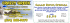### Lobsterfest Pete`s 12th Ann s 12th Ann s 12th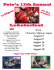### WRAP-IT-UP MEAL SOUTHERN-STyLE DRUMMERS MONSTER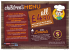### Doogan`s kiDs Menu - Doogan`s Of Aurora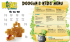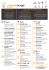### Best tasting Fries, Without The Oil!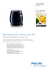### TEXAS TIC-TAC-TOE PERSONALIZE YOUR OWN WESTERN